# Basic Statistics Crash Course

A solid understanding of statistics is crucial for anyone who wants to work with data in data science, economics, psychology, social sciences, business, sports, etc.

But the terminology and calculations involved in statistics can be overwhelming for beginners. That’s why we created our Basic Statistics Crash Course that provides a simple example to help you understand fundamental statistical concepts, such as:

Our Basic Statistics Crash Course is perfect for preparing for university exams or enhancing your analytical skills. Soon, you’ll be well-equipped to tackle statistics questions during your data science interview or conduct analyses in your work or studies.

Watch our Statistics Crash Course video or read on for a quick introduction to the field.

## What Is Statistics?

Statistics concerns the collection, analysis, interpretation, and representation of data. Statisticians gather data through surveys, experiments, and observations. They form hypotheses and use statistical methods and models to test them, draw conclusions, and make predictions.

Statistics has numerous applications in science and business. It allows individuals and organizations to make informed decisions and derive data-driven insights. Any field that uses data involves statistics.

Our Basic Statistics Crash Course aims to help you grasp essential statistical concepts. We start with familiar terms like population and mean, then define the more complex-sounding (but still basic) ones like kurtosis and dispersion.

## Sample vs Population

To illustrate statistical concepts understandably, let’s provide an example.

Suppose a cooking class teacher asks all 36 students to complete a questionnaire at the beginning of the year. For various reasons, only 31 students submit their questionnaires. The teacher realized they would not have data on the entire class.

When the available data is limited, we say it’s a sample.

Had the teacher been able to collect data from all students in the class, then the data would be from the entire population.

A population includes every member of a group we’re interested in, while a sample is a smaller group taken from that population.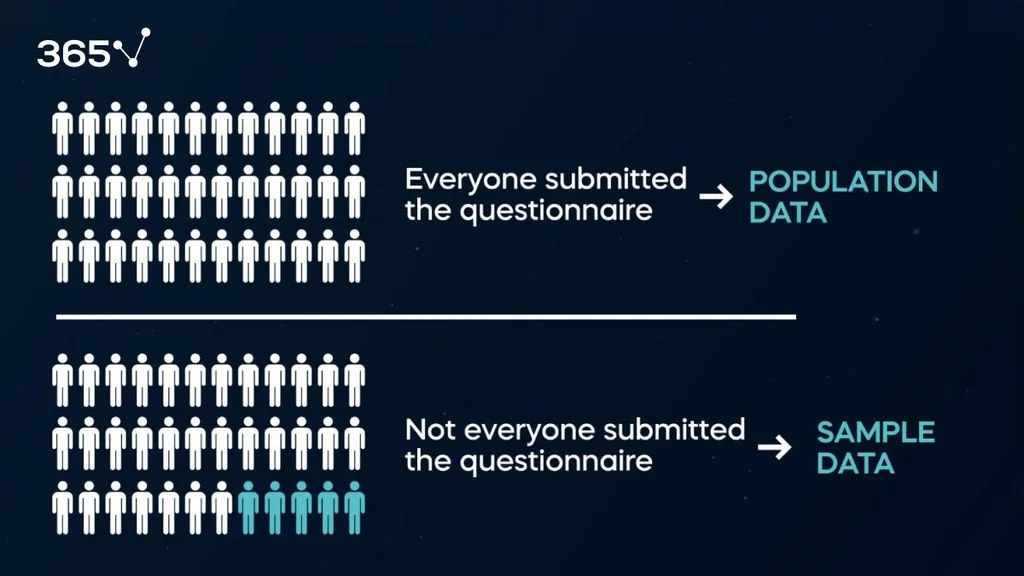Even though sometimes—as in this case—we cannot collect data for the entire population, sample data can be beneficial. They can allow us to make informed inferences about the whole population.

We’ll see how to do that later. But first, let’s define data types.

## Data Types

In the questionnaire, students were asked to provide two data types: numerical and categorical.

Numerical data are expressed in numbers, which can be measured—for example, students’ age, height, and weight. We can count, measure, and add years and kilograms because this is numerical information.

On the other hand, categorical data describe groups. A student’s preferred sport, gender, hair color, or eyes are categorical data. We can also consider Yes / No questions, such as “Does your family live within 1 kilometer from school?” categorical data. Adding or subtracting categorical data doesn’t make sense.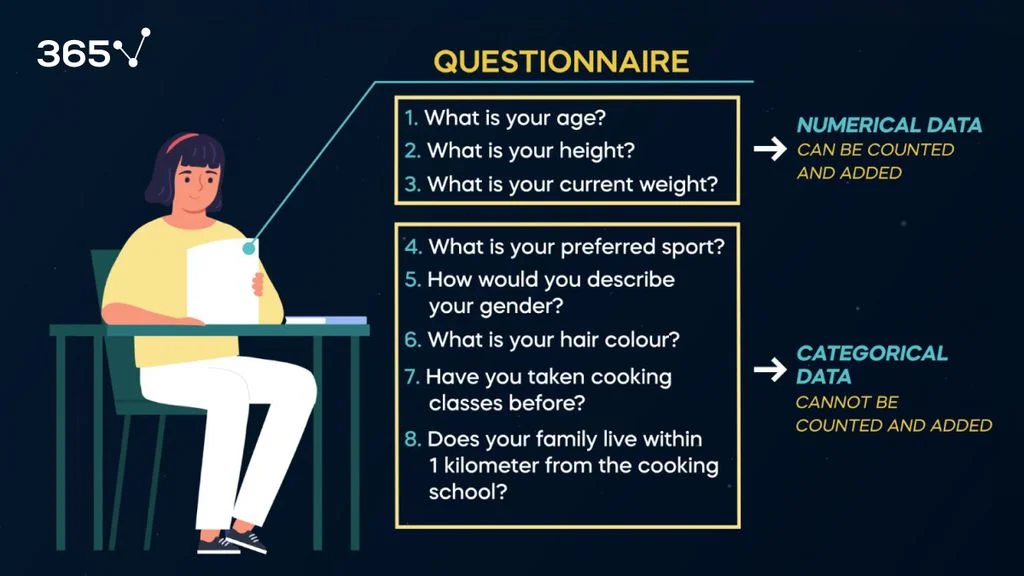## Measures of Central Tendency

We introduce the first statistical metrics in our Basic Statistics Crash Course: the measures of central tendency.

The measures of central tendency are mean, median, and mode. They give us an idea about the center of distribution or the typical value in a dataset.

This might sound more complex than it is. But these are basic concepts you encounter in statistics and everyday life.

Let’s go back to the example to understand them better.

Consider that the 31 students who submitted the questionnaire were asked to fill in their heights (numerical data that can be added).

Below are the student heights.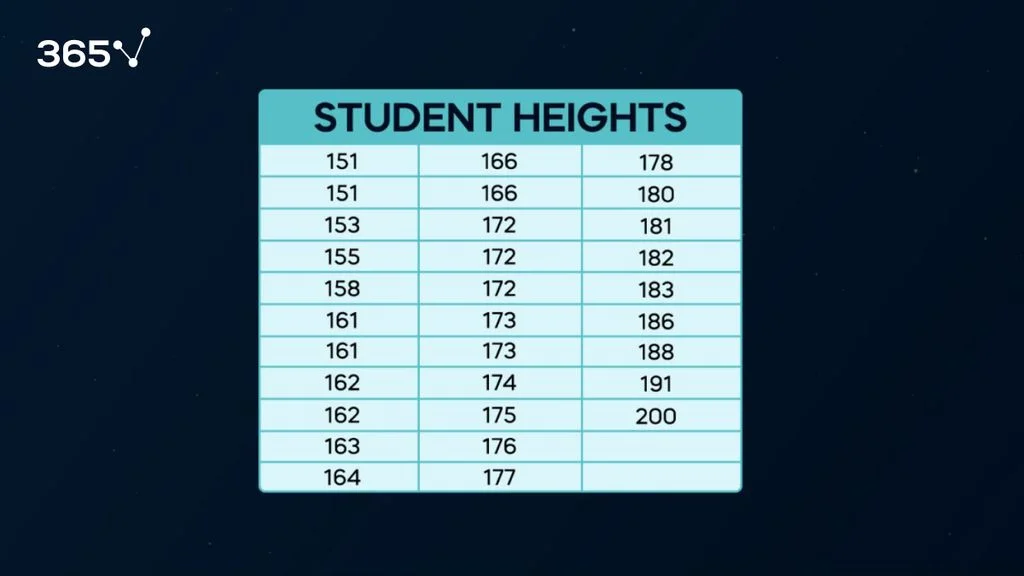First, let’s compute the mean (also called arithmetic average), which equals the sum of all heights divided by 31 (the number of students in our sample). We find that the average height of an individual is 171.2 centimeters.

Then, we have the median (the middle number in an ordered dataset). We organize the 31 heights in ascending order and take the middle number (16th number). So, the median is 172.

The mode is the value that occurs most often for numerical and categorical data. In our example, only one height is observed more than two times: 172. Therefore, this is the mode of our sample.

You can learn more about the measures of central tendency and compute them quickly with our mean, median, and mode calculator.

## Dispersion Measures

The average height is 171.2. But we also have students who are 191 and even 200 centimeters tall. So, we introduce dispersion measures—essential metrics in statistics.

Dispersion measures account for how observations spread out in a sample.

### Range

The range measures the difference between the highest and the lowest value. In our case, we obtain it by subtracting the shortest individual’s height from the tallest individual’s height (200 – 151 = 49 centimeters).

### Variance

A more popular and frequently used metric of dispersion is variance. Variance better represents the overall dispersion in a dataset because it considers an observation’s distance from the mean.

Depending on whether we work with population or sample data, we’ll need slightly different formulas.

Sample variance allows us to understand how data is spread out with respect to the sample mean. The sample formula is the unbiased estimator of the population formula.

$s^2=\sum_{i=1}^n \frac{(x_i-\bar{x})^2}{n-1}=\frac{(151-171.2)^2+(151-171.2)^2+(153-171.2)^2+(155-171.2)^2+\cdots+(200-171.2)^2}{31-1}= 147.3$

The formula for sample variance includes the sum of the squared difference between each individual’s height and the mean. Next, we divide the sum by the number of observations in the sample minus 1. We subtract 1 because we’re dealing with sample data, and this requires an adjustment.

If we were working with population data, we’d use the following formula.

$\sigma^2=\sum_{i=1}^{n}\frac{(X_i-\mu)^2}{n}$

Check out our variance calculator and adjacent article for a more detailed explanation and a quicker calculation.

### Standard Deviation

Once we have calculated the sample variance, we can quickly obtain the sample standard deviation, given by the square root of the variance.

$s=\sqrt{\sum_{i=1}^{n}\frac{{(X_i-\bar{X})}^2}{n-1}}$

Think of standard deviation as the average dispersion from the mean.

If the standard deviation is high, we deal with a dataset with highly spread-out observations. In contrast, a low standard deviation indicates the dataset observations are concentrated around the mean. In our case, the average dispersion from the mean is 12.1 centimeters.

When working with population data, we use the following formula:

$\sigma=\sqrt{\sum_{i=1}^{n}\frac{{(X_i-\mu)}^2}{n}}$

## Statistical Distributions

You can’t learn statistics without studying distributions.

Distributions reveal the overall shape of the data and show how frequently a value occurs in a dataset.

Shaped like a bell curve, the best-known and most widely used distribution is the normal distribution. Most of the data falls near the mean, with fewer values at the extremes. The mean is the highest point because it coincides with the mode. Observations are symmetrical on both ends. The normal distribution is beneficial because many real-life data follow this pattern.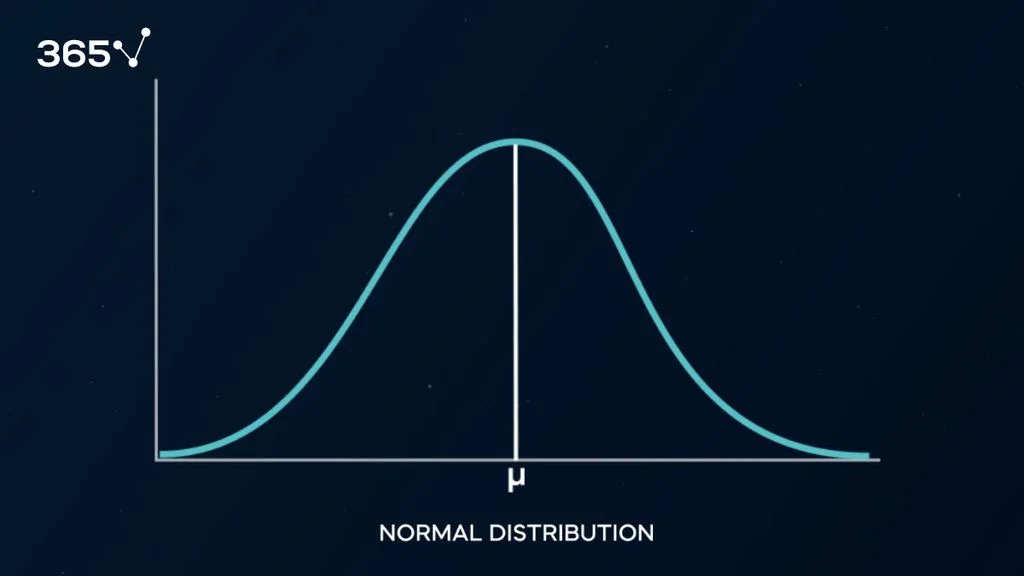Creating a histogram—one of the most popular data visualizations in statistics—using our students’ heights reveals that they are almost symmetrically distributed. Our graph approximates a normal distribution, but it is asymmetrical.

What do we call this?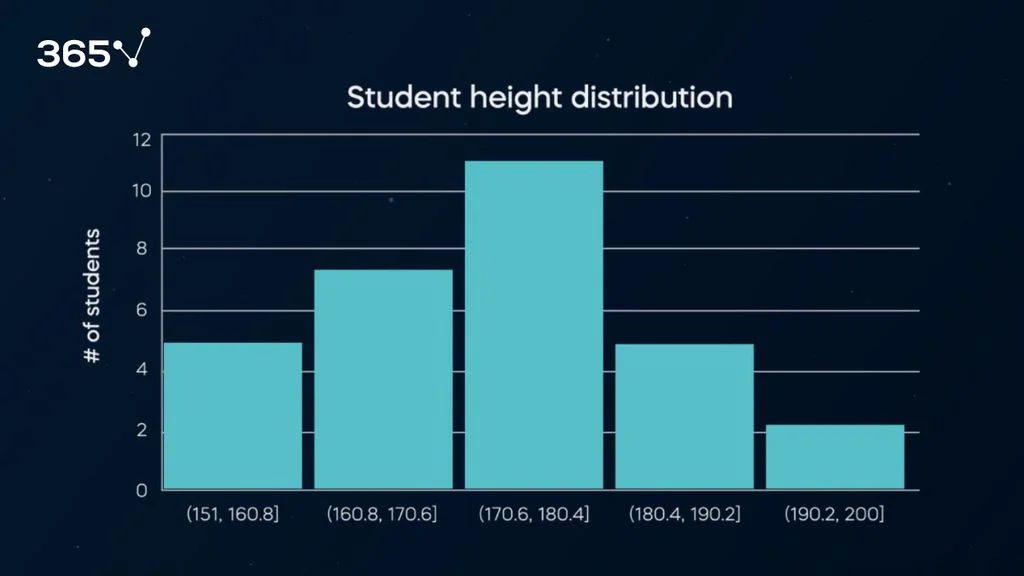### Skewness

If more observations in a dataset are concentrated on one side, we say the distribution is skewed.

Skewness is a measure of probability distribution asymmetry.

Positive skewness means a longer tail on the right side—that is, if we have had more students who are 190 or taller. Negative skewness means more observations (asymmetry) on the left side.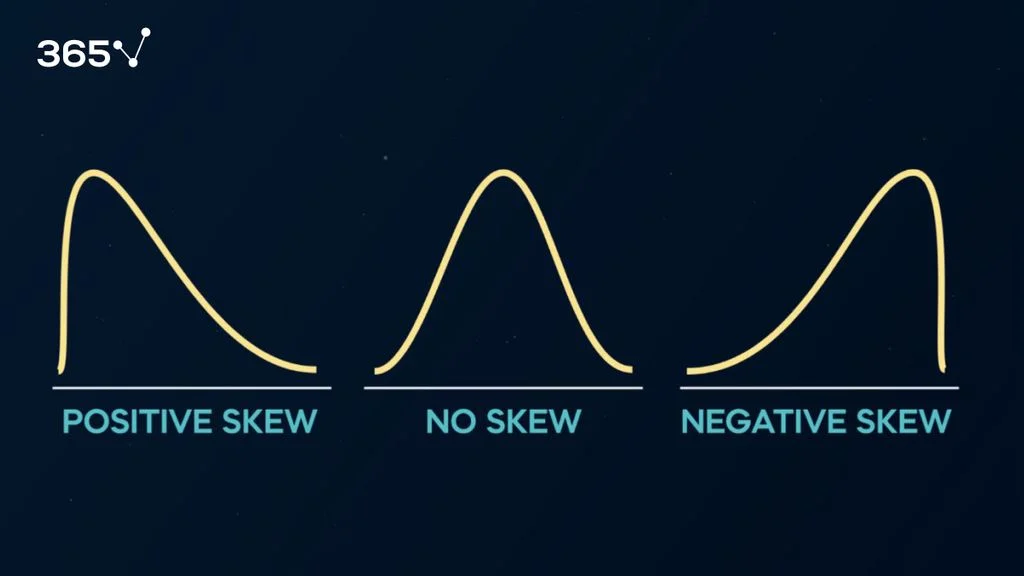In our case, the skewness is 0.23, which means a slightly longer right tail—largely thanks to the significant outliers of 191 and 200 centimeters. (We obtained this result with our skewness calculator.)

### Kurtosis

Another basic metric in statistics that helps us assess the shape of a probability distribution is kurtosis.

Kurtosis measures a distribution’s degree of tailedness. In other words, it shows how much of the data is in the tails compared to the center.

Based on the level of kurtosis, distributions can be:

• Leptokurtic: the tails are fatter compared to a normal distribution.
• Platykurtic: the tails are thinner compared to a normal distribution.
• Mesokurtic: the tails are the same as a normal distribution.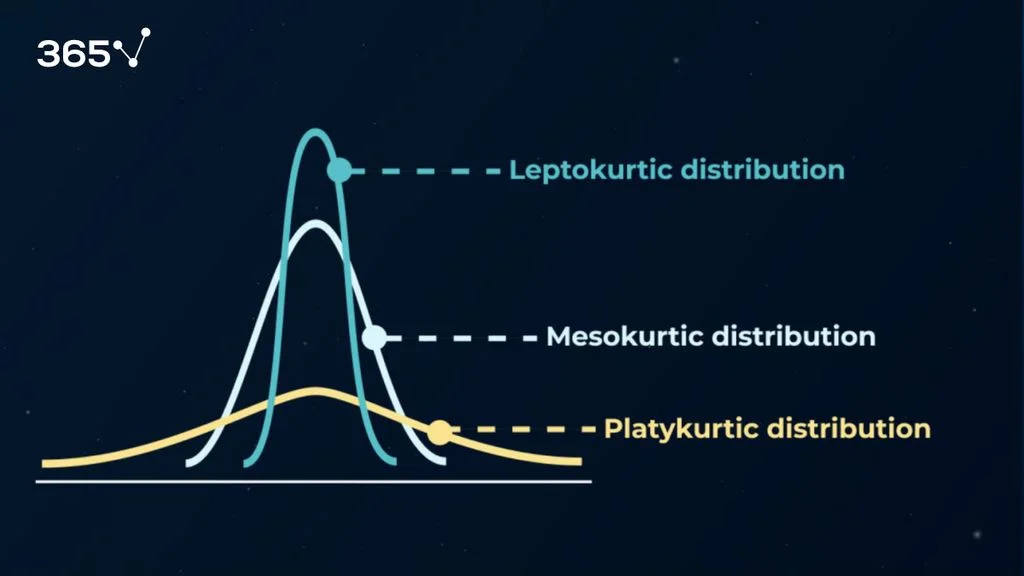In our case, the kurtosis is -0.3052, which means our distribution is platykurtic—indicating it’s relatively flat and with fewer outliers than a normal distribution. (We obtained this result with our kurtosis calculator.)

## Confidence Interval

Calculating the student’s mean height allows the teacher to form an expectation regarding how tall, on average, the students in the class are. Although this is convenient, the mean is a single number, or a point estimator.

And often, decision-makers feel uncomfortable working with a single number, which doesn’t give them information about dispersion. Confidence intervals solve this issue.

A confidence interval provides a range of possible realizations of the true population value. It’s an interval with which we are confident the population parameter will fall in 90, 95, or 99% of the cases.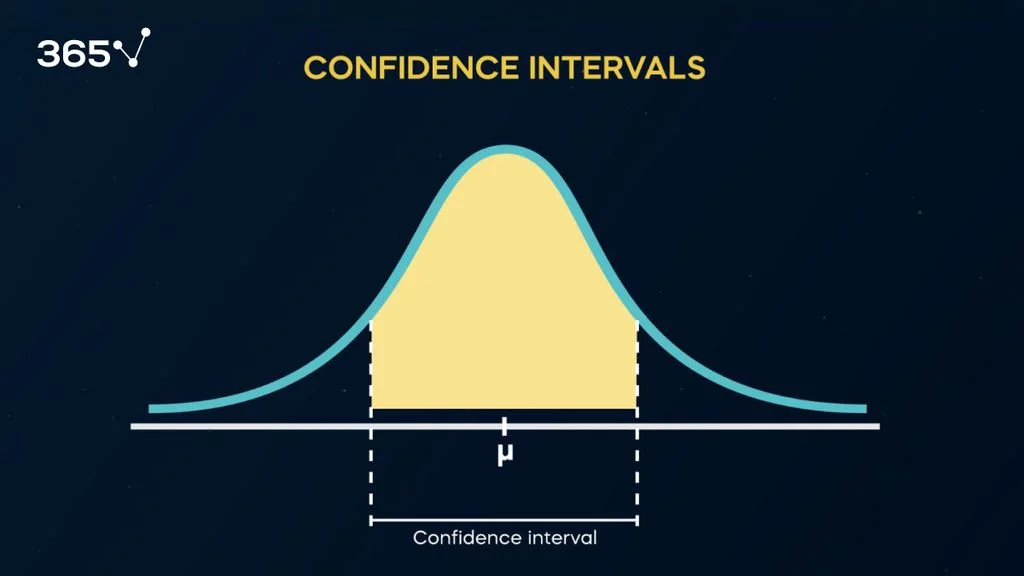In our case, the teacher wants to obtain a range that depicts the average height in 95% of the subjects rather than relying solely on the mean sample height.

We use the following confidence interval for our sample.

$\overline{X} \pm t_{(n-1),~\alpha/2} ~ \frac{s}{\sqrt{n}}$

The first component  of the formula is the sample mean we calculated earlier.

The second component is given by the product of t and the sample standard deviation divided by the square root of the number of observations. We call this term the Standard Error.

Here, we already know the sample standard deviation and the number of observations. But what is t?

Because the normal distribution is perfectly symmetrical, statisticians have been able to create two statistical tables with values. One table (the z-table) is for when the population variance is known, and another (the t-table) is used when the population variance is unknown. Both tables calculate the area under the curve considering the number of observations and the degree of confidence we want to have.

The degree of confidence will vary if we opt for 90, 95, or 99% certainty.

Let’s see how this works in practice.

In our case, we must use a t-distribution table because we deal with sample data. If we opt for 95% probability, we’ll leave a 2.5% probability of estimation error on each side. Considering that we have 31 observations (30 degrees of freedom because we have n-1), we obtain the t-value equal to 2.042. This allows us to compute the margin of error.

Then, we add and subtract the margin of error to obtain our confidence interval. With a 95% degree of certainty, the average height of students falls in the range of 166.1 to 176.2 centimeters.

In this case, our population variance and standard deviation are unknown, so we use the t-statistic.

But here’s something your Introduction to Statistics class may not have taught you.

For sufficiently large samples—even if the population variance is unknown—we can use the z-table. The rule of thumb is always to use the t-table with less than 30 observations. The result after 30 observations would be very close, so you can use either the t- or z-table.

## Hypothesis Testing

Hypothesis testing is statistics 101. It uses the same calculation mechanics and logic as confidence intervals but reframes the problem slightly differently.

Let’s get back to our example.

Say the teacher’s intuition was that the average height of all students was 170 centimeters. How would we test if this estimate is correct?

The first step is to form two hypotheses: the null hypothesis (denoted H0) and the alternative hypothesis (marked with H1). The null hypothesis is the assumption we want to test, and the alternative is everything else.

In our example, the null hypothesis indicates that the mean student height equals 170 centimeters, while the alternative shows that the mean student height is not 170 centimeters.

To perform the test, we must check if 170 centimeters is close to the sample’s true mean. If it is, we fail to reject the null hypothesis. Otherwise, we reject the null hypothesis.

The concept of the null hypothesis is similar to “innocent until proven guilty.”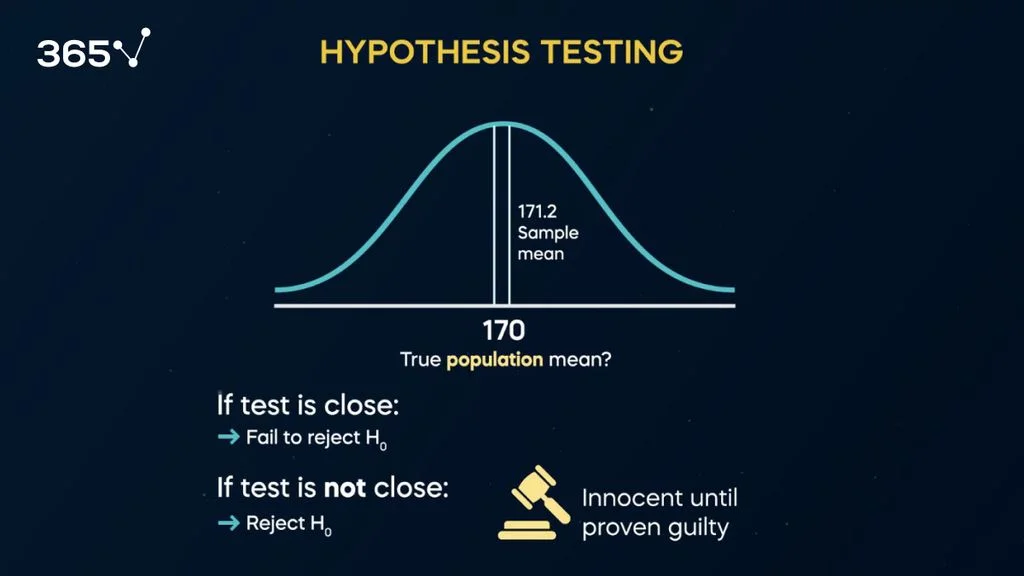We assume the mean height is 170 centimeters (H0) and try to prove otherwise. We can have a height lower than 170 or higher than 170. The null hypothesis can be rejected if either of the two is correct, so we say this is a two-sided test.

We use the following formula:

$t = \frac{\overline{X} - \mu}{\frac{s}{\sqrt{n}}}$

Looks familiar to what we had before, doesn’t it?

This time we solve for t by subtracting 170 from the sample mean and dividing by the standard deviation divided by the square root of the number of observations. We obtain the t statistic, which is equal to 0.5.

The next step is to compare the t statistic with a value from the t-distribution table determined based on a pre-selected (95%) confidence level, remembering that we have 31 – 1 = 30 observations.

Using a t-table, we find the critical t-value for a two-tailed test with 30 degrees of freedom and a 95% confidence level is 2.042.

Since the absolute value of our t statistic (0.5) is less than the critical t-value (2.042), we can conclude that our result is not statistically significant at the 95% confidence level. In other words, we fail to reject the null hypothesis that the average student height is not 170 centimeters.

This wraps up our Basic Statistics Crash Course. What’s next?

## Next Steps

At 365 data science, we love statistics and want to help you learn the fundamentals. With our Statistics course, you can take your skills to the next level. Check it out as a follow-up to this article.

And if statistics is your stepping stone to a data science or analytics career, you’ve come to the right place. We offer structured career tracks that help you become a job-ready data scientist or data analyst.Start your career